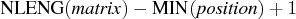Previous Page | Next Page

 Language Reference

## SUBSTR Function

takes substrings of matrix elements

SUBSTR( matrix, position<, length>)

The inputs to the SUBSTR function are as follows:
matrix
is a character matrix or quoted literal.

position
is a numeric matrix or scalar giving the starting position.

length
is a numeric matrix or scalar giving the length of the substring.
The SUBSTR function takes a character matrix as an argument along with starting positions and lengths and produces a character matrix with the same dimensions as the argument. Elements of the result matrix are substrings of the corresponding argument elements. Each substring is constructed by using the starting position supplied. If a length is supplied, this length is the length of the substring. If no length is supplied, the remainder of the argument string is the substring.

The position and length arguments can be scalars or numeric matrices. If position or length is a matrix, its dimensions must be the same as the dimensions of the argument matrix or submatrix. If either one is a matrix, its values are applied to the substringing of the corresponding elements of the matrix. If length is supplied, the element length of the result is MAX(length); otherwise, the element length of the result isThe following statements return the output shown:
```
B={abc def ghi, jkl mno pqr};
a=substr(b,3,2);
```

```
A        2 rows      3 cols    (character, size 2)

C  F  I
L  O  R
```
The element size of the result is 2; the elements are padded with blanks.Previous Page | Next Page | Top of Page